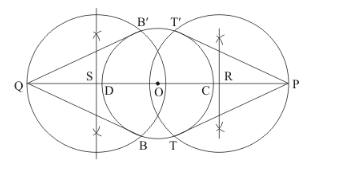# Draw a circle of radius 3 cm. Take two points P and Q on one of its extended

Question:

Draw a circle of radius 3 cm. Take two points P and Q on one of its extended diameter each at a distance of 7 cm from its centre. Draw tangents to the circle from these two points P and Q.

Solution:

Given that

Construct a circle of radius, andextended diameter each at distance of 7cm from its centre. Construct the pair of tangents to the circle from these two points.

We follow the following steps to construct the givenStep of construction

Step: I- First of all we draw a circle of radius $=3 \mathrm{~cm}$.

Step: II- Make a line $C D=$ diameter $-6 \mathrm{~cm}$.

Step: III-Extend the line $C D$ in such a way that point $C P=D Q=7 \mathrm{~cm}$

Step: IV-CP at a distance of $O P=7+3=10 \mathrm{~cm}$, and join $O P$ draw a right bisector of $O P$, intersecting $O P$ at $R$.

Step V:- Similarly, $D Q$ at a distance of $O Q=7+3=10 \mathrm{~cm}$, and join $O Q$ draw a right bisector of $O Q$, intersecting $O Q$ at $S$.

Step VI: Taking R and S as centre and radius $O S=O R$, draw a circle to intersect the given circle at $T$ and $T$ '

B and B ’respectively.

Step: VII- Joins PT and PT’ as well as QB and QB’ to obtain the require tangents.

Thus,are the required tangents.